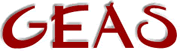[ < ] [ > ] [ << ] [ Up ] [ >> ] [Top] [Contents] [Index] [ ? ]

### 2.3.7 Bit functions

Sometimes it's desirable to store lots of simple `on/off'-type information. The quick and dirty approach is then to allocate one integer for each of this information bearers and use them to hold either a one or a zero to indicate the state. This makes for easy access and easy understanding, but it's a pain when you want to store the info and it takes a lot of memory.

Instead you can use strings where every bit in a character (8 per char) can hold an information state of the on/off kind. The max number of bits right now in a string is something like 1200 = a string length of 150 characters. However, I doubt you'll ever need to store that many states.

You set the bits with the efun `set_bit()` which takes two arguments, the first is the string that actually contains the bits and the second is an integer specifying exactly what bit you want to set. Remember that the first bit is bit number 0. To clear a bit you use the efun `clear_bit()` that works analogous to `set_bit()`. When you need to test a bit you use the efun `test_bit()` which simply takes the same arguments as the other efun but returns 1 or 0 depending on whether the tested bit was set or not.

You don't have to allocate a string in advance when you use `set_bit()`. Both `set_bit()` and `clear_bit()` return the new modified string, and in case it's not wide enough it will be extended by `set_bit()`. However, `clear_bit()` will not shorten it automatically.

 ```string set_bit(string bitstr, int the_bit) string clear_bit(string bitstr, int the_bit) int test_bit(string bitstr, int the_bit) e.g. // Set bit 23 in a new bitfield. string bf; bf = ""; bf = set_bit(bf, 22); // Clear bit 93 in the same bitfield bf = clear_bit(bf, 92); // Test bit 3 if (test_bit(bf, 2)) write("Set!\n"); else write("Clear!\n"); ```

 [ < ] [ > ] [ << ] [ Up ] [ >> ] [Top] [Contents] [Index] [ ? ]

This document was generated by Ronny Wikh on July, 8 2003 using texi2html